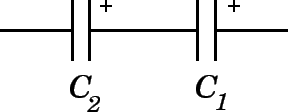# Combination Capacitor Circuits

## Key Questions

• For capacitors in parallel:The total capacitance C_(TOT is given by:

${C}_{T O T} = {C}_{1} + {C}_{2}$

Since ${C}_{T O T} = \frac{Q}{V}$ the total can be found from $Q = {C}_{T O T} V$ where V is the voltage drop across the capacitors.

For capacitors in series:$\frac{1}{C} _ \left(T O T\right) = \frac{1}{C} _ 1 + \frac{1}{C} _ 2$

So $Q = {C}_{T O T} V$

• Series, parallel and combinations of series and parallel/There are four examples of combinations in the diagram. The following points show how to calculate the total capacitance of each combination.
1. Series
The equivalent capacitance, C , of the combination is worked out as follows:

$\frac{1}{C} = \frac{1}{C} _ 1 + \frac{1}{C} _ 2 + \frac{1}{C} _ 3$
or
$C = \frac{1}{1 / {C}_{1} + 1 / {C}_{2} + 1 / {C}_{3}}$

Total capacitance decreases in series.

2. Parallel
$C = {C}_{1} + {C}_{2} + {C}_{3}$

Total capacitance increases in parallel.

3. "Parallel in series"

$\frac{1}{C} = \frac{1}{C} _ 1 + \frac{1}{{C}_{2} + {C}_{3}}$

4. "Series in parallel"

$C = \frac{1}{1 / {C}_{1} + 1 / {C}_{2}} + {C}_{3}$

Example with numbers based on combination 4.

${C}_{1} = 200 \mu F$, ${C}_{2} = 400 \mu F$, ${C}_{3} = 400 \mu F$

$C = \frac{1}{1 / \left(200 \cdot {10}^{- 6}\right) + 1 / \left(400 \cdot {10}^{- 6}\right)} + \left(400 \cdot {10}^{- 6}\right)$

⇒C=1/7500+400*10^-6=133*10^-6+400*10^-6

$C = 533 \mu F$